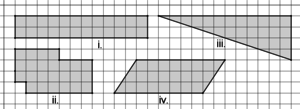# 24 Unit Squares

Alignments to Content Standards: 6.G.A.1The area of each figure shown is 24 square units.

1. On grid paper, find a way to draw more figures with an area of 24 square units. Draw at least one of each:
1. A rectangle that is different from the one shown.
2. A polygon with more than 4 sides that is different from the one shown.
3. A right triangle that is different from the one shown.
4. A parallelogram.
2. For each of the figures you draw, explain how you know its area is exactly 24 square units.

## IM Commentary

The purpose of this activity is to help students think a little more flexibly about the concept of area before studying, generally, the areas of triangles and special quadrilaterals. Tasks that ask "What is the area of the given figure?" lend themselves to using formulas without understanding. However, "Create a figure with a given area" is appealing because it requires students to understand the meaning of area. Drawings produced by students can offer many opportunities for classroom discussion and for students to explain their reasoning (MP 3).

Before students start the task, they may benefit from a brief discussion of what is meant by "area." Teachers may ask and open up for discussion questions like, "What does it mean to say that the areas are 24 square units? 24 whats? Where are the 24 things in this figure that belong to this measurement of area?" Answering these questions for the rectangle is straightforward, but for the right triangle will require more reasoning. Some valid approaches are shown.In the figure on the left, it is shown that the triangle is exactly 1/2 of a rectangle whose area is 48 unit squares. Therefore, the area of the triangle must be 24 square units. In the figure in the middle, a section of the right triangle is lopped off and relocated, creating a rectangle whose area is 24 unit squares. The figure on the right shows how students might match up pieces of unit squares to create complete unit squares. This matching-up process can be continued for a total of 24 unit squares. (Note: the visual confirmation that the two pieces look like they combine to make a square is sufficient. Proving such is beyond the scope at this grade level.)

Some students may benefit from the availability of scissors and large grid paper. That way, they can cut and rearrange shapes. This can be useful for justifying areas but also constructing figures of a given area.

## Solution

Answers will vary. Some possible responses are shown.Students may take one of several valid approaches to explaining how they know an area is 24 square units. Surrounding with a rectangle, chopping off and rearranging pieces, or matching up pieces of individual unit squares are some examples. These approaches are shown more fully in the commentary.